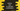# How to calculate combination in Python## Python combination :

The combination is the selection of set of elements from a collection, without regard to the order. For example, for the numbers 1,2,3, we can have three combinations if we select two numbers for each combination : (1,2),(1,3) and (2,3).

In python, we can find out the combination of the items of any iterable. For that, we need to use the itertools package. Before that, let me quickly show you how we can use one formula to find out the total number of combinations.

### Combination formula :

If we have n distinct elements and if we are taking r elements at a time, we can have the below amount of combinations :

``nCr``

This is equal to :

``n!/r!(n-r)!``

For example, if we have 3 elements and if we are taking 2 elements at a time, we will have 3!/2!(3-2)! or 3 combinations.

### Python itertools combinations :

combinations function is defined in python itertools library. We need to import it whenever we want to use combinations.

It provides two different functions. One is to find out the combinations without replacement, and another is to find out with a replacement. Following are the definitions of these functions :

``````combinations(it, r)

combinations_with_replacement(it, r)``````

The first one will find out the combinations of length r from the iterable it. It will not include any repeated elements.

The second one,combinationswithreplacement will find the combinations of length r from the iterable it with repeated elements.

### Example of combinations() and combinationswithreplacement() with a string :

Let’s consider the below example:

``````import itertools as it

print(list(it.combinations('abc', 2)))
print(list(it.combinations_with_replacement('abc', 2)))``````

If you execute it, it will print the below output :

``````[('a', 'b'), ('a', 'c'), ('b', 'c')]
[('a', 'a'), ('a', 'b'), ('a', 'c'), ('b', 'b'), ('b', 'c'), ('c', 'c')]``````

The second print statement results in three extra pairs with repeated elements.

### Example of combinations() with a list :

Let’s take a look at the below example:

``````import itertools as it

given_list = [1, 2, 3]
list_combinations = it.combinations(given_list, 2)

for item in list(list_combinations):
print(item)``````

It will print the below output :

``````(1, 2)
(1, 3)
(2, 3)``````

### Example of combination() with a list with duplicate elements :

If we have duplicate elements in the list like below :

``````import itertools as it

given_list = [1, 1, 3]
list_combinations = it.combinations(given_list, 2)

for item in list(list_combinations):
print(item)``````

It will print the below output :

``````(1, 1)
(1, 3)
(1, 3)``````

Because, the items are picked based on their position, not on their values.

If we use the above example with combinations_with_replacement, it will print:

``````(1, 1)
(1, 1)
(1, 3)
(1, 1)
(1, 3)
(3, 3)``````

### Example of combinations() with a tuple :

The below example uses a tuple and finds all combinations :

``````import itertools as it

given_tuple = ('one', 'two', 'three')
tuple_combinations = it.combinations(given_tuple, 2)

for item in list(tuple_combinations):
print(item)``````

It will print :

``````('one', 'two')
('one', 'three')
('two', 'three')``````

### Example of combinations() with a dictionary :

We can also use combinations() with a python dictionary. For example:

``````import itertools as it

given_dictionary = {
'a': 'one',
'b': 'two',
'c': 'three'
}
dictionary_combinations = it.combinations(given_dictionary, 2)

for item in list(dictionary_combinations):
print(item)``````

It returns the combinations of keys of the dictionary :

``````('a', 'b')
('a', 'c')
('b', 'c')``````

Using these keys, we can access the values of the dictionary.